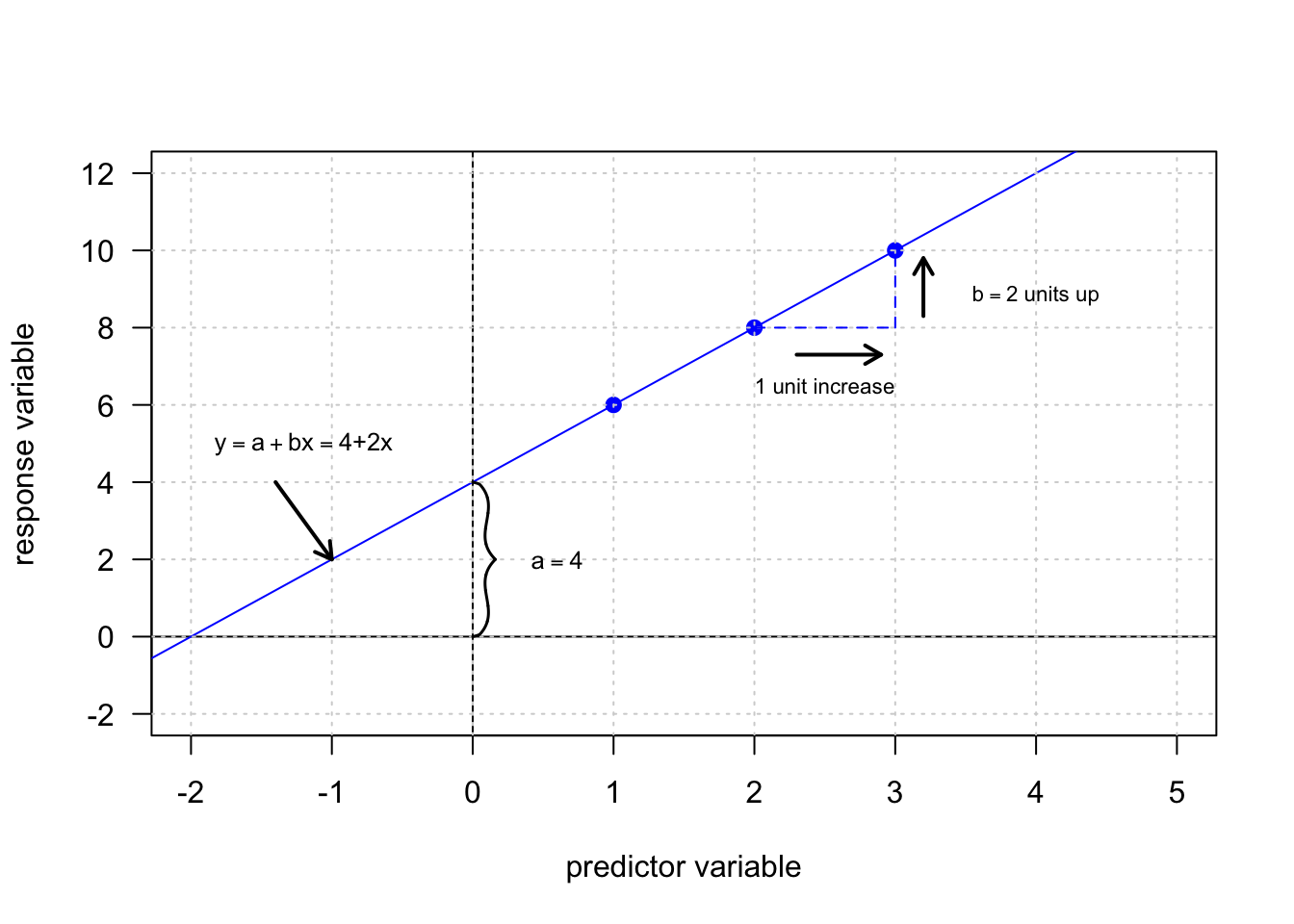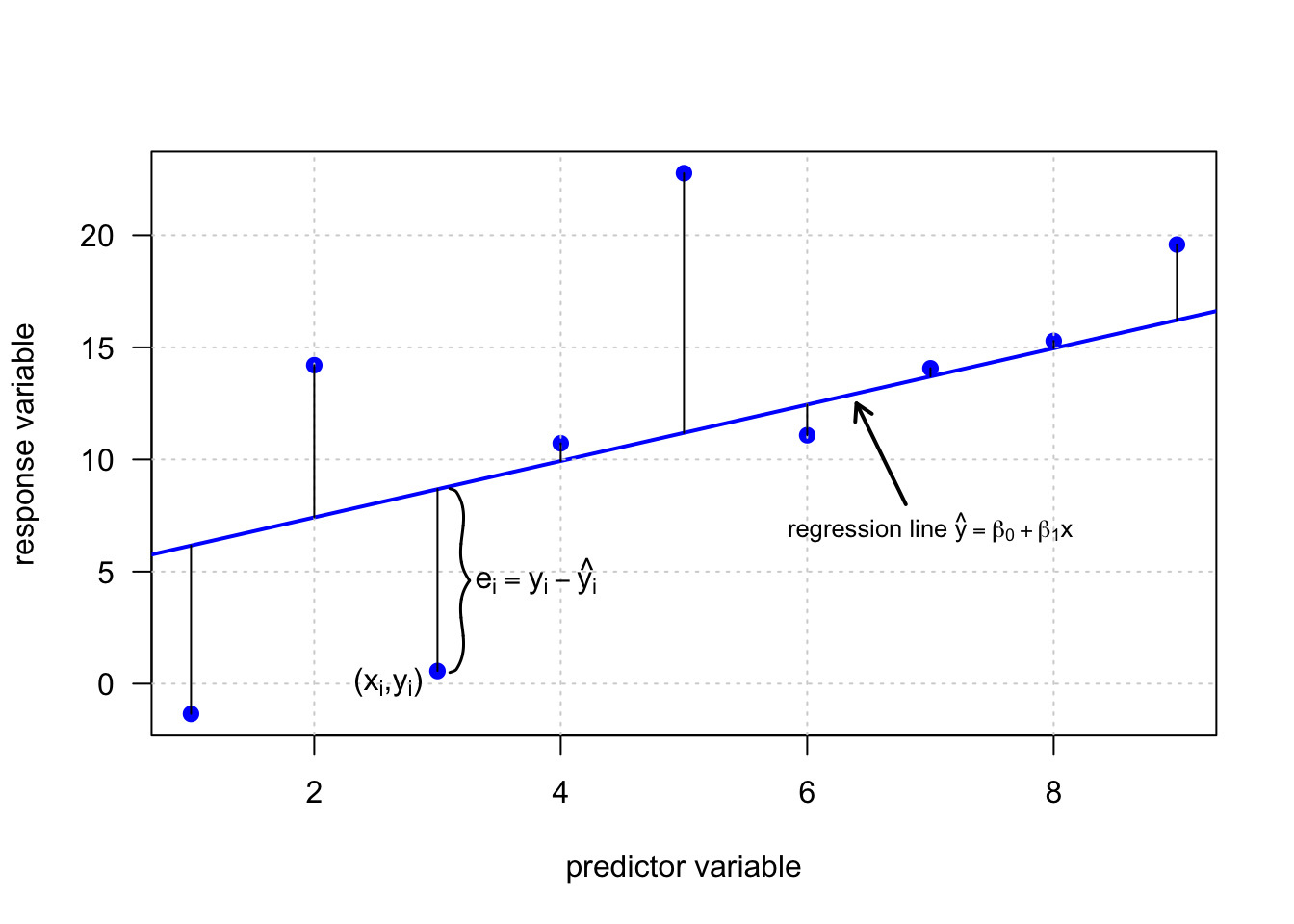In this section we discuss a special type of regression, which is called simple linear regression. In this special case of regression analysis the relationship between the response variable $$y$$ and and the predictor variable $$x$$ is given in form of a linear equation

$y= a + bx\text{,}$

where $$a$$ and $$b$$ are constants. The number $$a$$ is called intercept and defines the point of intersection of the regression line and the $$y$$-axis ($$x=0$$). The number $$b$$ is called regression coefficient. It is a measure of the slope of the regression line. Thus, $$b$$ indicates how much the $$y$$-value changes when the $$x$$-value increases by 1 unit. The adjective simple refers to the fact that the outcome variable is related to a single predictor. The model is considered as a deterministic model, as it gives an exact relationship between $$x$$ and $$y$$.

Let us consider a simple example. Given a population of $$n = 3$$ points with cartesian coordinates $$(x_i,y_i)$$ of $$(1,6)$$, $$(2,8)$$ and $$(3,10)$$. These points plot on a straight line and thus, can be described by a linear equation model in the form of $$y= a + bx$$, where the intercept $$a=4$$ and $$b=2$$.In many cases however, the relationship between two variables $$x$$ and $$y$$ is not exact. This is due to the fact, that the response variable $$y$$ is affected by other unknown and/or random processes, that are not fully captured by the predictor variable $$x$$. In such a case the data points do not line up on a straight line. However, the data still may follow an underlying linear relationship. In order to take these unknowns into consideration a random error term, denoted by $$\epsilon$$, is added to the linear model equation, thus resulting in a probabilistic model in contrast to the deterministic model from above.

$y = a + b x + \epsilon$

where the error term $$\epsilon_i$$ is assumed to consist of independent normal distributed values, $$e_i \sim N(0, \sigma^2)$$.

In linear regression modelling following assumptions are made about the model (Mann 2012).

• The random error term $$\epsilon$$ has a mean equal to zero for each $$x$$.
• The errors associated with different observations are independent.
• For any given $$x$$, the distribution of errors is normal.
• The distribution errors for each $$x$$ has the same (constant) standard deviation, which is denoted by $$\sigma_{\epsilon}$$.

Let us consider another example. This time we take a random sample of sample size $$n = 8$$ from a population. In order to emphasis that the values of the intercept and slope are calculated from sample data, $$a$$ and $$b$$ are denoted by $$\beta_0$$ and $$\beta_1$$, respectively. In addition, the error term $$\epsilon$$ is denoted as $$e$$. Thus, $$\beta_0$$, $$\beta_1$$ and $$e$$ are estimates based on sample data for the population parameters $$a$$, $$b$$ and $$\epsilon$$.

$\hat y = \beta_0 + \beta_1 x + e \text{,}$

where $$\hat y$$ is the the estimated or predicted value of $$y$$ for any given value of $$x$$.The error $$e_i$$ for each particular pair of values ($$x_i,y_i$$), also called residual, is computed by the difference of the observed value $$y_i$$ and the predicted value given by $$\hat y_i$$.

$e_i = y_i - \hat y_i$

Depending on the data $$e_i$$ is a negative number if $$y_i$$ plots below the regression line or it is a positive number if $$y_i$$ plots above the regression line.